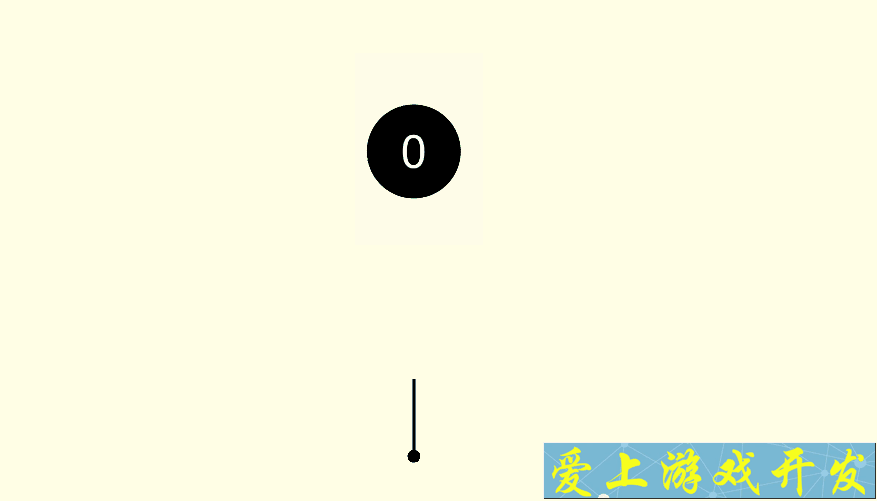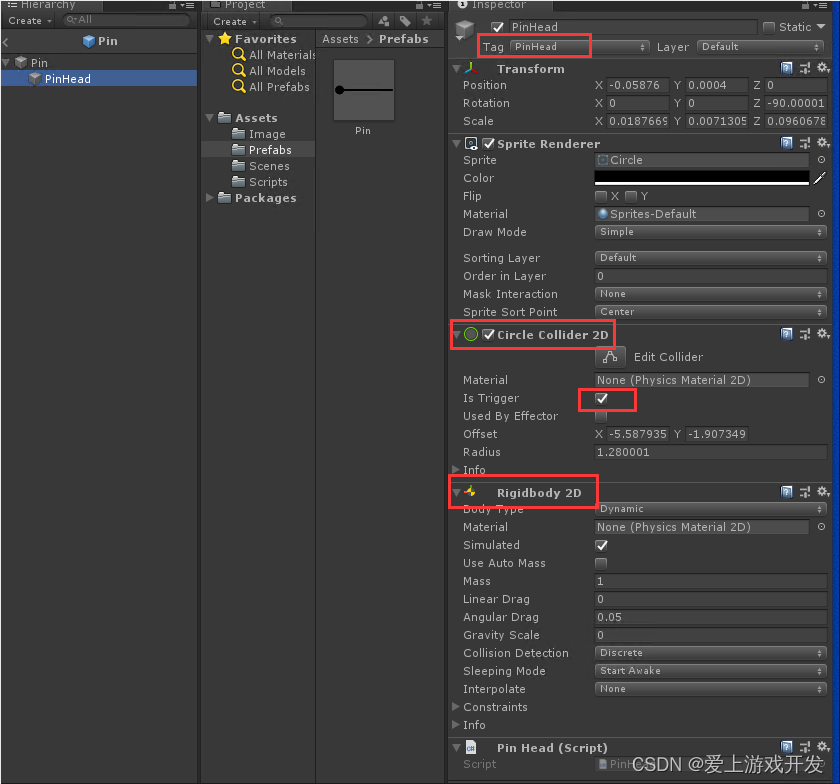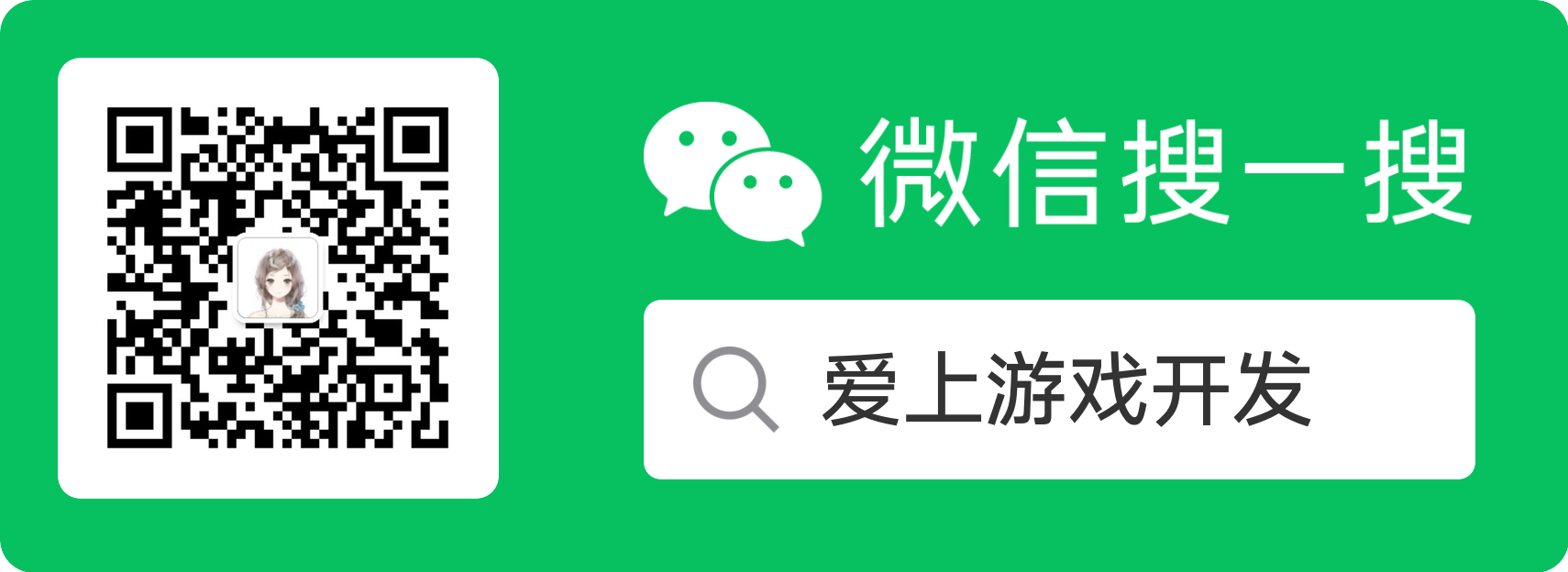## Unity实战之见缝插针

|     |   0 浏览1.圆盘的转动。
2.动态生成需要被插的针。
3.针的移动。
4.针的旋转(与转盘旋转同步)。
5.游戏得分。
6.游戏成功与失败的判断。

## 圆盘的转动

``````public float speed = 90;
transform.Rotate(new Vector3(0, 0, speed * Time.deltaTime));
``````

``````using System.Collections;
using System.Collections.Generic;
using UnityEngine;

public class RotateSelf : MonoBehaviour {

public float speed = 90;

// Update is called once per frame
void Update () {
transform.Rotate(new Vector3(0, 0, -speed * Time.deltaTime));
}
}
``````

## 实例化针

1.刚进入游戏时，场景只有一个圆盘，下方需要实例化针。
2.当玩家发射出针后，针飞到圆盘上，下方又需要实例化。

``````public GameObject pinPrefab;
private Transform spawnPoint;-- 实例化位置

void Start () {
spawnPoint = GameObject.Find("SpawnPoint").transform;
}

void SpawnPin()
{
GameObject.Instantiate(pinPrefab, spawnPoint.position, pinPrefab.transform.rotation);
}
``````

``````void Start () {
SpawnPin();
}
``````

``````private void Update()
{
if (Input.GetMouseButtonDown(0))
{
SpawnPin();
}
}
``````

## 针移动至圆盘

``````private bool isFly = false;
private bool isReach = false;
``````

``````// GameManager.cs
void SpawnPin()
{
currentPin = GameObject.Instantiate(pinPrefab, spawnPoint.position, pinPrefab.transform.rotation).GetComponent<Pin>();
}
``````
``````// GameManager.cs
private void Update()
{
if (Input.GetMouseButtonDown(0))
{
currentPin.StartFly();
SpawnPin();
}
}
``````
``````// Pin.cs
public void StartFly()
{
isFly = true;
isReach = true;
}
``````

``````// Pin.cs
private Transform startPoint;
private Vector3 targetCirclePos;

void Start () {
startPoint = GameObject.Find("StartPoint").transform;
}

void Update () {
if (isFly == true)
{
if (isReach == false)
{
transform.position = Vector3.MoveTowards(transform.position, startPoint.position, speed * Time.deltaTime);
if (Vector3.Distance(transform.position, startPoint.position) < 0.01f)
{
isReach = true;
}
}
}
else
{
transform.position = Vector3.MoveTowards(transform.position, targetCirclePos, speed * Time.deltaTime);
if(Vector3.Distance( transform.position,targetCirclePos) < 0.01f)
{
transform.position = targetCirclePos;
isFly = false;
}
}
}
``````

## 针的旋转

``````// Pin.cs
private Transform circle;
transform.parent = circle;
``````

## 游戏得分

``````// GameManager.cs
public Text scoreText;
private int score = 0;

score++;
scoreText.text = score.ToString();
``````

## 失败成功判定

#### 产生碰撞的条件

1.若要产生碰撞，必须双方都要有碰撞器。
2.运动的一方一定要有刚体，另一方有无刚体无所谓。

#### 接触的两种方式

1.Collision碰撞，造成物理碰撞，可以在碰撞时执行OnCollision事件。
2.Trigger触发，取消所有的物理碰撞，可以在触发时执行OnTrigger事件。

#### Collision碰撞

（1）双方都有碰撞体
（2）运动的一方必须有刚体
（3）双方不可同时勾选Kinematic运动学。
（4）双方都不可勾选Trigger触发器

### Trigger触发

（1）双方都有碰撞体
（2）运动的一方必须是刚体
（3）至少一方勾选Trigger触发器

2.添加Rigibody 2D组件``````//PinHead.cs
using System.Collections;
using System.Collections.Generic;
using UnityEngine;

public class PinHead : MonoBehaviour {

private void OnTriggerEnter2D(Collider2D collision)
{
{
//游戏结束
}
}
}
``````

### 游戏结束处理

``````// GameManager.cs
private bool isGameOver = false;
public void GameOver()
{
if (isGameOver) return;
GameObject.Find("Circle").GetComponent<RotateSelf>().enabled = false;
isGameOver = true;
}
``````

``````if (isGameOver) return;
``````

-----------------------------